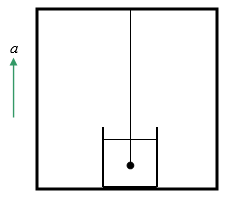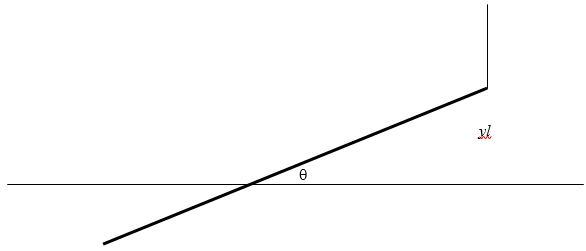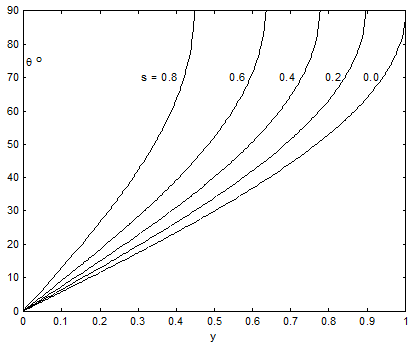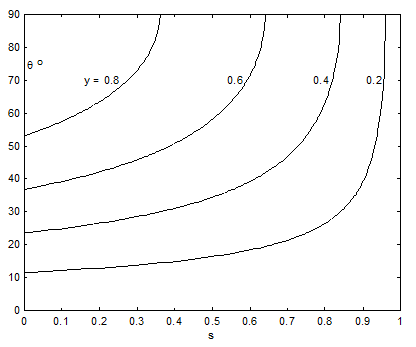$$\require{cancel}$$

# 16.8: Some Simple Examples

•• Contributed by Jeremy Tatum
• Emeritus Professor (Physics & Astronomy) at University of Victoria

As we pointed out in the introduction to this chapter, this chapter is less demanding than some of the others, and indeed it has been quite trivial so far. Just to show how easy the topic is, here are a few quick examples.

Example $$\PageIndex{1}$$

A cylindrical vessel of cross-sectional area A is partially filled with water. A mass $$m$$ of ice floats on the surface. The density of water is $$\rho_{0}$$ and the density of ice is $$\rho$$. Calculate the change in the level of the water when the ice melts, and state whether the water level rises or falls.

Example $$\PageIndex{2}$$

A cork of mass $$m$$, density $$\rho$$, is held under water (density $$\rho_{0}$$) by a string. Calculate the tension in the string. Calculate the initial acceleration if the string is cut.Example $$\PageIndex{3}$$

A lump of lead (mass $$m$$, density $$\rho$$) is held hanging in water (density $$\rho_{0}$$) by two strings as shown. Calculate the tension in the strings.Example $$\PageIndex{4}$$

A hydrometer (for our purposes a hydrometer is a wooden rod weighted at the bottom for stability when it floats vertically) floats in equilibrium to a depth $$z_{1}$$ in water of density $$\rho_{1}$$. If salt is added to the water so that the new density is $$\rho_{2}$$, what is the new depth $$z_{2}$$?Example $$\PageIndex{5}$$

A mass $$m$$, density $$\rho$$, hangs in a fluid of density $$\rho_{0}$$ from the ceiling of an elevator (lift). The elevator accelerates upwards at a rate $$a$$. Calculate the tension in the string.Example $$\PageIndex{6}$$

A hydrometer of mass $$m$$ and cross-sectional area $$A$$ floats in equilibrium do a depth $$h$$ in a liquid of density $$\rho$$. The hydrometer is then gently pushed down and released. Determine the period of oscillation.

Example $$\PageIndex{7}$$

A rod of length $$l$$ and density $$s\rho$$($$s<1$$) floats in a liquid of density $$\rho$$. One end of the rod is lifted up through a height $$yl$$ so that a length $$xl$$ remains immersed. I have drawn it with the rope vertical. Must it be?)i. Find $$x$$ as a function of $$s$$.

ii. Find $$\theta$$ as a function of $$y$$ and $$s$$.

iii. Find the tension $$T$$ in the rope as a function of $$m,\ g$$ and $$s$$.

Draw the following graphs:

a. $$x$$ and $$\frac{T}{(mg)}$$ versus $$s$$.

b. $$\theta$$ versus $$y$$ for several $$s$$.

c. $$\theta$$ versus $$s$$ for several $$y$$.

d. $$x$$ versus $$y$$ for several $$s$$.

e. $$\frac{T}{(mg)}$$ versus $$y$$ for several $$s$$.

1. No, it does not.

2. $$T=\left(\frac{\rho_{0}-\rho}{\rho}\right)mg$$

3. $$T_{1}=\frac{\left(\frac{\rho-\rho_{0}}{\rho}\right)mg}{\cos\theta_{1}+\frac{\sin\theta_{1}}{\tan\theta_{2}}}$$ $$T_{2}=\frac{\left(\frac{\rho-\rho_{0}}{\rho}\right)mg}{\cos\theta_{2}+\frac{\sin\theta_{2}}{\tan\theta_{1}}}$$

4. $$z_{2}=\frac{\rho_{1}}{\rho_{2}}z_{1}$$

5. $$T=m\left[a+g\left(\frac{\rho-\rho_{0}}{\rho}\right)\right]$$

6. $$P=2\pi\sqrt{\frac{m}{\rho Ag}}$$

7.

1. $$x=1-\sqrt{1-s}$$
2. $$\sin\theta=\frac{y}{\sqrt{1-s}}$$
3. $$T=mg\left(\frac{\sqrt{1-s}-(1-s)}{s}\right)$$

1.2.3.4.5.